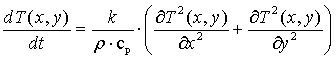# Calculus Without Tears

### Derivation of the differential equation model for 2-D heat transfer

Fourier's heat transfer law in 2-D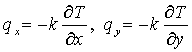It states that the heat flux qx in the x direction is proportional to negative of the partial derivative of temperature w.r.t. x, and the heat flux qy in the y direction is proportional to the negative of the partial derivative of temperature w.r.t. y.

Flux is a flow rate divided by an area, so that flux∙area equals a flow rate. A flow of what? Heat energy.

Since flux is proportional to the negative of the directional derivative, heat energy flows from a region with high temperature to a region with low temperature.

Given a thin plate, the control volume, as in the diagram, the heat flux through the surface A is given by the –k times the directional derivative of T in x direction evaluated at the center of A, (x-dx/2,y), where we assume that the control volume is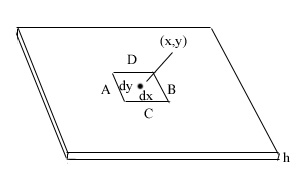small enough that the temperature can be considered as constant on a side, and thus the rate heat is flowing into the small square through surface A is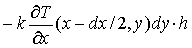Note that a positive q represents heat flowing to the right and hence into the control volume through side A.

Similarly, the rate heat is flowing out of the square thru side B is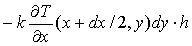The rate heat is flowing into the square thru side C is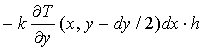The rate heat flowing out of the square thru side D is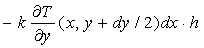Adding these four terms gives the rate that heat energy is flowing in/out from all sidesGrouping terms and dividing by the volume gives the rate that heat energy is flowing into the square per unit volume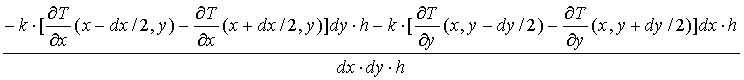Heat energy flowing in/out of the control volume changes its temperature. The relationship between heat energy and temperature is given by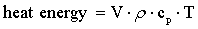Thus the rate of change of temperature in the control volume is given by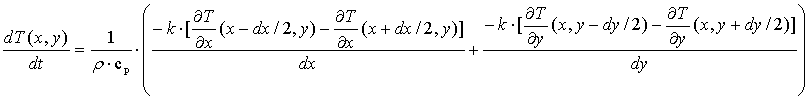and letting dx -> 0, dy -> 0, we have the differential equation model for time dependent 2-D heat transfer